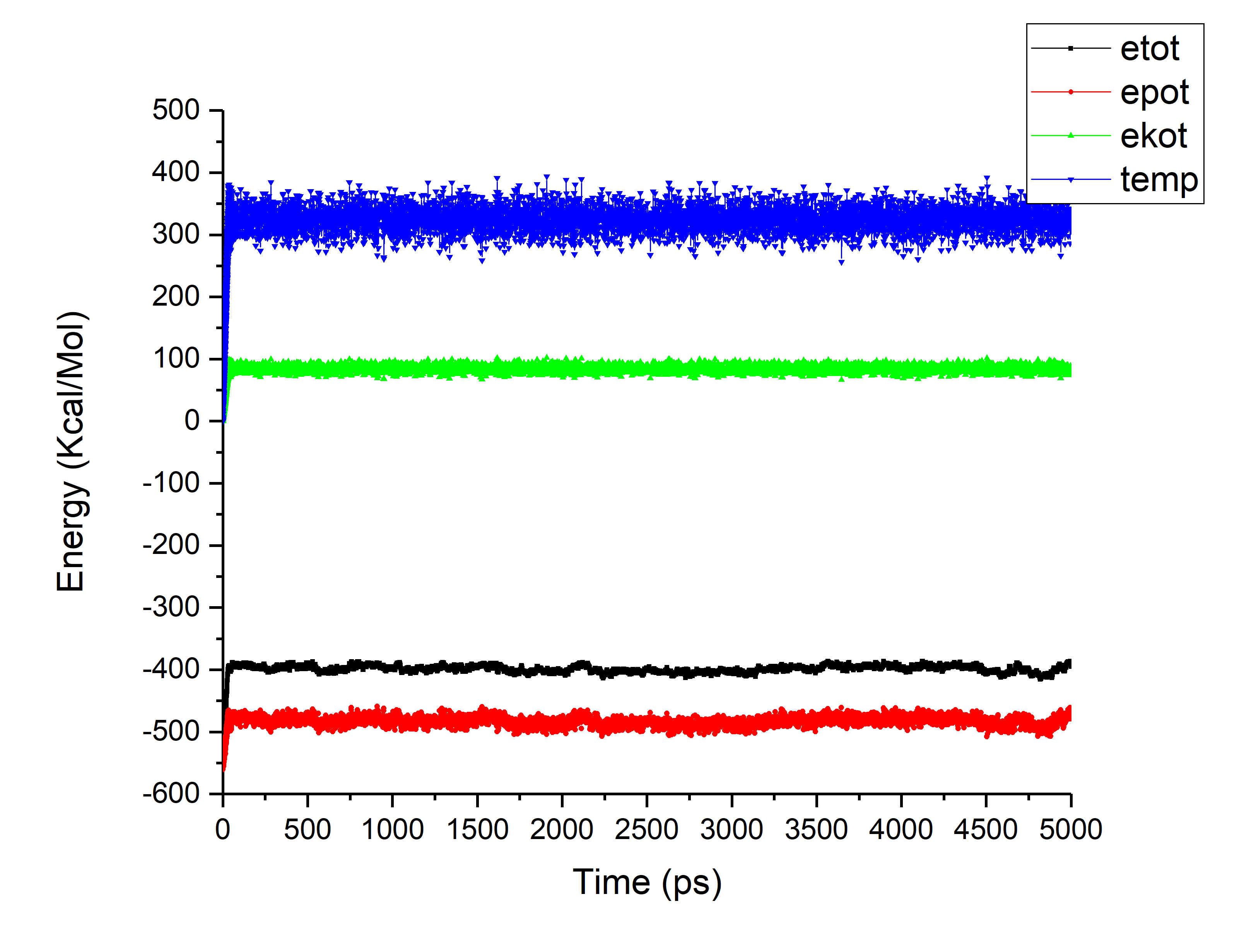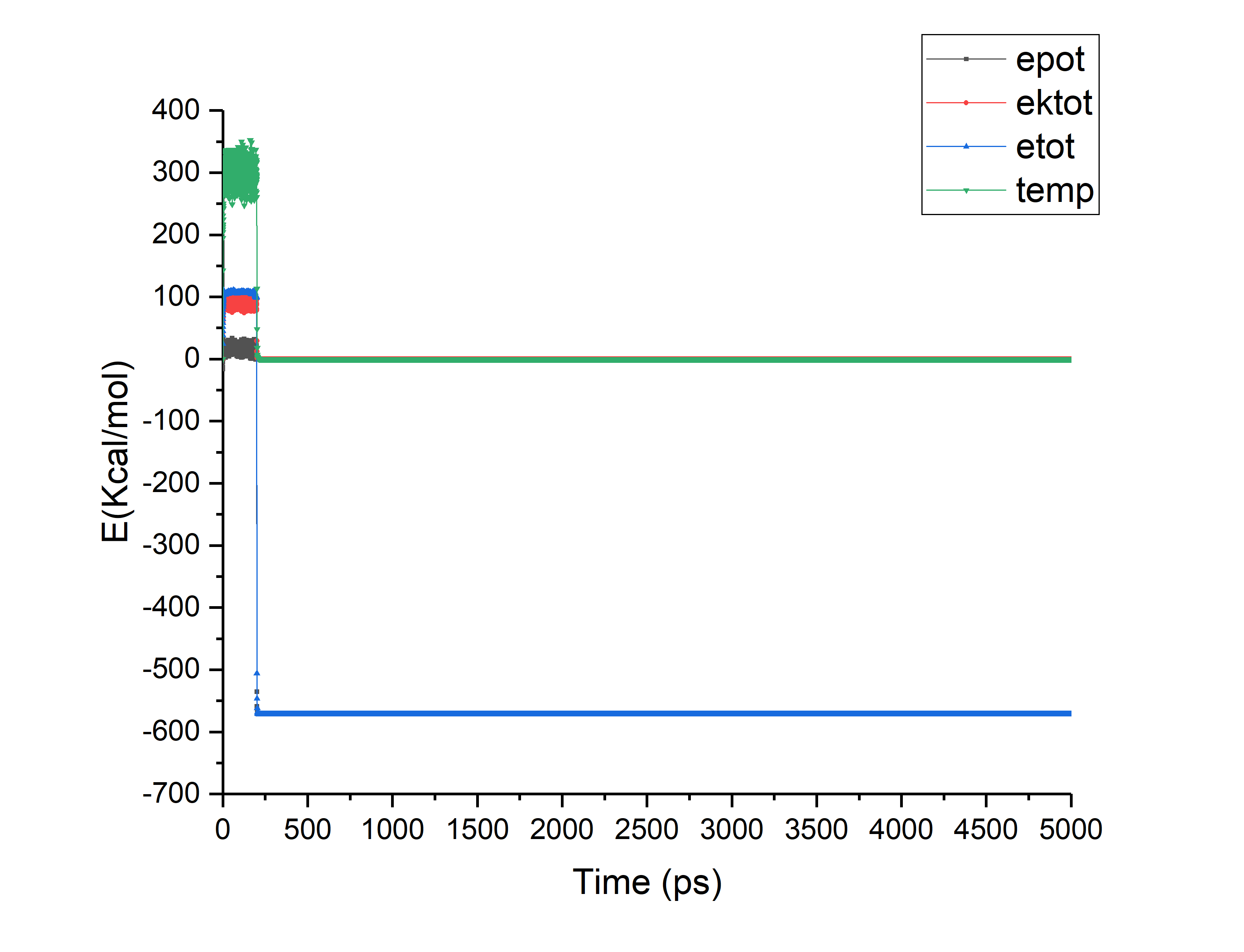[AMBER] Choosing a temperature schedule for Simulated annealing

From: Rajbinder Kaur Virk <rajbinderkaurvirk.gmail.com>
Date: Thu, 29 Nov 2018 12:49:39 +0530

Respected Amber users
I intend to find low energy structure of a small metalloprotein conjugate
through simulated annealing method of AMBER. I am a beginner and currently
struggling at most of the steps.
I followed two protocols, Input files and the results of both protocols has
been attached with this email.
In first case (protocol 1) the annealing was performed till 325 K for 50
psec thereafter equilibration was done at 325 K itself for 50 nanosec (in
batches).
Stage 1 heating of TC5b 300 to 325K
&cntrl
imin=0, irest=1, ntx=5,
nstlim=40000, dt=0.0005,
ntc=2, ntf=2,
ntt=1, tautp=1.0,
tempi=300.0, temp0=325.0,
ntpr=50, ntwx=50,
ntb=0, igb=1,
cut=999.,rgbmax=999.
/
In the second case, the temperature was increased till 600 k, to access
maximum conformational space and then cooling was done till 0 K. Thereafter
equilibration was done till 5 nano sec at 0 K. Unfortunately the
temperature of the system neither reached uptill 600 and neither decreased
to 0. It remained at arround 350 K.
Can you please guide me about the mistake that I might be doing in the
second case.
&cntrl

nstlim=200000, pencut=-0.001, nmropt=0,
ntpr=200, ntt=1, ntwx=200,
cut=999.,ntb=0, vlimit=10,
rgbmax=999.
/
&wt type='TEMP0', istep1=0,istep2=50000,value1=600.,
value2=600., /
&wt type='TEMP0', istep1=50001, istep2=180000, value1=600.0,
value2=100.0, /
&wt type='TEMP0', istep1=180001, istep2=200000, value1=0.0,
value2=0.0, /

&wt type='TAUTP', istep1=0,istep2=50000,value1=0.4,
value2=0.4, /
&wt type='TAUTP', istep1=50001,istep2=180000,value1=4.0,
value2=4.0, /
&wt type='TAUTP', istep1=180001,istep2=200000,value1=1.0,
value2=1.0, /
&wt type='TAUTP', istep1=180001,istep2=200000,value1=0.1,
value2=0.05, /

&wt type='REST',
istep1=0,istep2=150000,value1=0.1,
value2=1.0, /
&wt type='REST',
istep1=150001,istep2=200000,value1=1.0,
value2=1.0, /

&wt type='END' /
LISTOUT=POUT

DISANG=RST

Also, can you please suggest some reading material to clear my concepts in
order to decide an annealing schedule.
Thank you
with regards
Rajbinder Kaur Virk
```--
*With regards*
*Rajbinder Kaur Virk*
*Research Scholar *
*Department of Biophysics*
*Panjab University *
*Chandigarh-160014*

```

_______________________________________________
AMBER mailing list
AMBER.ambermd.org
http://lists.ambermd.org/mailman/listinfo/amber(image/png attachment: Protocol1.png)(image/png attachment: Protocol2.png)

Received on Wed Nov 28 2018 - 23:30:04 PST
Custom Search